Courses

# Measurement of Power, Energy, and Power Factor Notes | EduRev

## Electrical Engineering (EE) : Measurement of Power, Energy, and Power Factor Notes | EduRev

The document Measurement of Power, Energy, and Power Factor Notes | EduRev is a part of the Electrical Engineering (EE) Course Electrical and Electronic Measurements.
All you need of Electrical Engineering (EE) at this link: Electrical Engineering (EE)

Electric Power Measurements

1. DC Electric Circuits
In order to identify an electrical system in DC, a measurement of the electrical current, I, flowing through the bipoles and their electric voltage, V, across the terminals has to be formed.
These measurements can be arranged by means of a DC ammeter and voltmeter. The electric power, P, related to such a bipole, can be defined as the product of the voltage and current applied to: P =V × I . The power generated by an active bipole can

2. Single-Phase AC Electric Circuits
The electric power in a single-phase AC circuit can be defined as:
p(t) = v(t)i(t)
where p, v, i are instantaneous power, voltage, and current, respectively.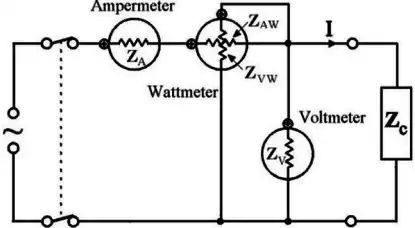the instantaneous power is
p =  v.i   =  2 V I cos wt . cos (wt  - φ)
The average power is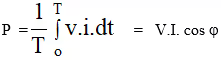where V and I are the effective (R.M.S.) values of the voltage and current.
Voltage & Current Relationship in 3-Phase Circuit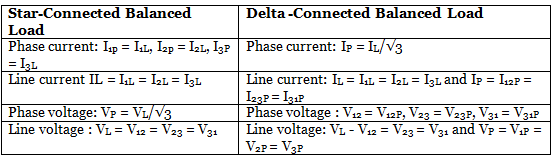3. Three Phase Power Measurement using Two Watt-meter
Figure below shows the Two Watt-meter connection may be used to determine the power in a three-phase three-wire circuit (for unbalanced condition).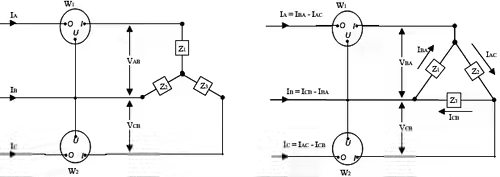Star connection:
Power indicated by W1 :
P1 = VAB IA cosΦ
Power indicated by W2 :
P2 = VCB IC cosΦ
Power indicated by W2 :
P2 =  VCB IC cosΦ
ΦCB-C is the phase difference between VCB and IC. VCB  = VCN - VBN (Potential drop across W2).
Sum of the powers measured by the two wattmeters W1 and W2 would equal:
PT = P1 + P2

4. Three Phase Power Measurement – Analysis in the Balanced Case
Phase difference between VAB and VAN is 30°.
If the load is assumed to be inductive, the current is lagging behind their respective phase voltage by Φ, the phase difference between IA and VAB is = (30°+ Φ).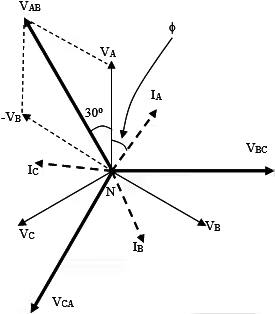• For a balanced system the magnitudes VAB = VCB = VL (line voltage:voltage between any pair of terminals, eg. VAB).

For a balanced supply and three-phase load:
Power indicated by Watt-meter W1:
P1 = VabIa cosΦab-a =  VL.IL.cos(Φ+30o)
where VL is the magnitude of the line voltage and IL that of the line current.
Power indicated by wattmeter W2:
P2 = VcbIc cosΦcb-c =  VL.IL.cos(Φ - 30o)
The sum of the two Wattmeter readings:
P1 + P2 = VL.IL.cos(Φ +30o) + VL.IL.cos(Φ-30o) = VL.IL.[cos(Φ+30o) + cos(Φ-30o)]
= VL IL cosΦ

• This is the total power PT consumed by the load. Hence, the sum of the readings of the two meters gives the total power PT consumed by the load.
• In this method, the reading of the Wattmeter W1 can become negative if f is greater than 60o.

5. To Solve for Power Factor from the above analysis Consider P= W1 & P2 = W2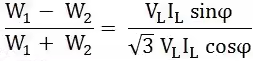or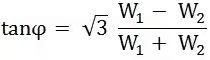or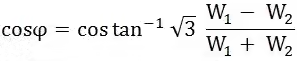by using above formula we can easily calculate the condition of Power Factor.

Measurement of Power Factor

An AC system of Active power (P), Reactive power (Q), and Apparent power (S) plays a major role in electric power technology.
The terms of Active power, Reactive power, and Apparent power are applied to steady-state alternating current circuits in which the voltages and currents are non-sinusoidal.
Today it is characteristic in most parts of the applications that the current and voltage are Non-Sinusoidal.

1. Definition of Power Factor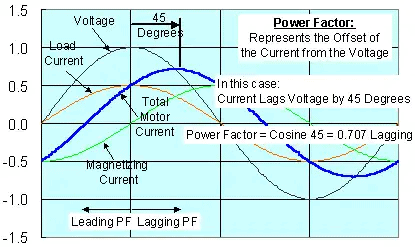In the earlier definition the power factor is the classical definition, for pure sine wave current and voltage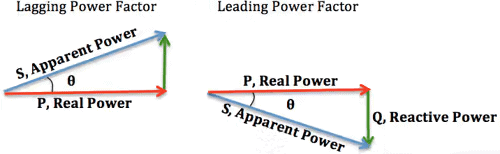• The power factor is basically the “angle cosine of that lagging current". In simple words, current is lagging by voltage with some angle and if we take the cosine of that angle we will get power factor.
• Now how to get that lagging angle? This is only problem left here. If somehow we can measure the time difference of both waveforms then we can find our required angle by using the formula below: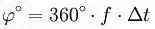• Where frequency (f) is the frequency of the system which may be 50 or 60 Hz.

2. Zero Cross Detection

• Zero cross detection is a method which can enable us to measure the time between voltage and current.
• In this technique we get a high value whenever a zero will cross the system.
• There are many ways to implement it. But remember, this technique is the heart of this project so implementation must be accurate.
• In this project we implemented zero crossing using LM358 an 8 pin IC having dual amplifiers in it.
• In zero crossing, we have to get a “high” value during crossing of zero in waveforms. So to get that value we use amplifier as a comparator which will  compare the non inverting reference value and then act accordingly.

3. Definition for non-sinusoidal current and sinusoidal voltage of power factor is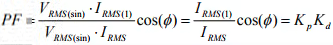• The relationship between the Total Harmonic Distortion and Distortion Factor can be derived from the following expressions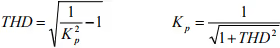• The formula for the Power Factor by means of the Total Harmonic Distortion with zero phase shift between the voltage and the fundamental component of the current is given by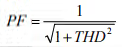Measurement of Energy

Energy, heat, work and power are four concepts that are often confused. If force is exerted on an object and moves it over a distance, work is done, heat is released (under anything other than unrealistically ideal conditions) and energy is transformed. Energy, heat and work are three facets of the same concept. Energy is the capacity to do (and often the result of doing) work.

• The SI unit of energy, heat and work is the joule (J). The British thermal unit (Btu) or one of its multiples; and the kilowatt hour (kWh).
• Power is the rate at which work is done (or heat released, or energy converted).
• A light bulb draws 100 joules of energy per second of electricity, and uses that electricity to emit light and heat (both forms of energy). The rate of one joule per second is called a watt. The light bulb, operating at 100 J/s, is drawing power of 100 Watts.

1. Energy meter or watt hour meter is classified in accordance with several factors such as

• Type of display like analog or digital electric meter.
• Type of metering point like grid, secondary transmission, primary and local distribution.
• End applications like domestic, commercial and industrial.
• Technical like three phases, single phase, HT, LT and accuracy class meters.
2. Electro-Mechanical induction type Energy meter
• It is the popularly known and most common type of age old watt hour meter.
• It consists of rotating aluminum disc mounted on a spindle between two electro magnets.
• Speed of rotation of disc is proportional to the power and this power is integrated by the use of counter mechanism and gear trains.
• It comprises of two silicon steel laminated electromagnets i.e., series and shunt magnets.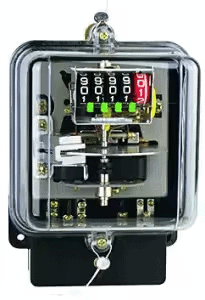3. Electronic Energy Meters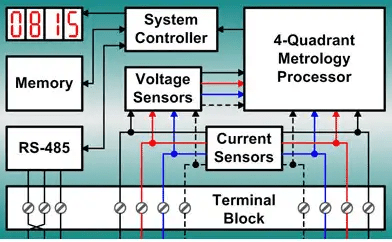• These are of accurate, high procession and reliable types of measuring instruments as compared to conventional mechanical meters.
• It consumes less power and starts measuring instantaneously when connected to load.
• These meters might be analog or digital.
• In analog meters, power is converted to proportional frequency or pulse rate and it is integrated by counters placed inside it.
• In digital electric meter power is directly measured by high end processor.
• The power is integrated by logic circuits to get the energy and also for testing and calibration purpose. It is then converted to frequency or pulse rate.
4. Smart Energy Meters

It is an advanced metering technology involving placing intelligent meters to read, process and feedback the data to customers. It measures energy consumption, remotely switches the supply to customers and remotely controls the maximum electricity consumption. Smart metering system uses the advanced metering infrastructure system technology for better performance.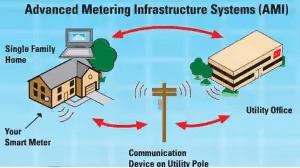• These are capable of communicating in both directions.
• They can transmit the data to the utilities like energy consumption, parameter values, alarms, etc and also can receive information from utilities such as automatic meter reading system, reconnect/disconnect instructions, upgrading of meter software’s and other important messages.

5. Formula to calculate the amount of Energy consumed by a Load
The calculation is as follows:
[number of  hours’ use] x [number of days’ use] x ([capacity of appliance expressed in watt] / 1,000) = number of kWh
The capacity should be divided by 1,000 to convert the number of watts into the number of kilowatts. This finally gives us the number of kWh (kilowatt-hours).

Offer running on EduRev: Apply code STAYHOME200 to get INR 200 off on our premium plan EduRev Infinity!

7 docs|6 tests

,

,

,

,

,

,

,

,

,

,

,

,

,

,

,

,

,

,

,

,

,

,

,

,

,

,

,

;